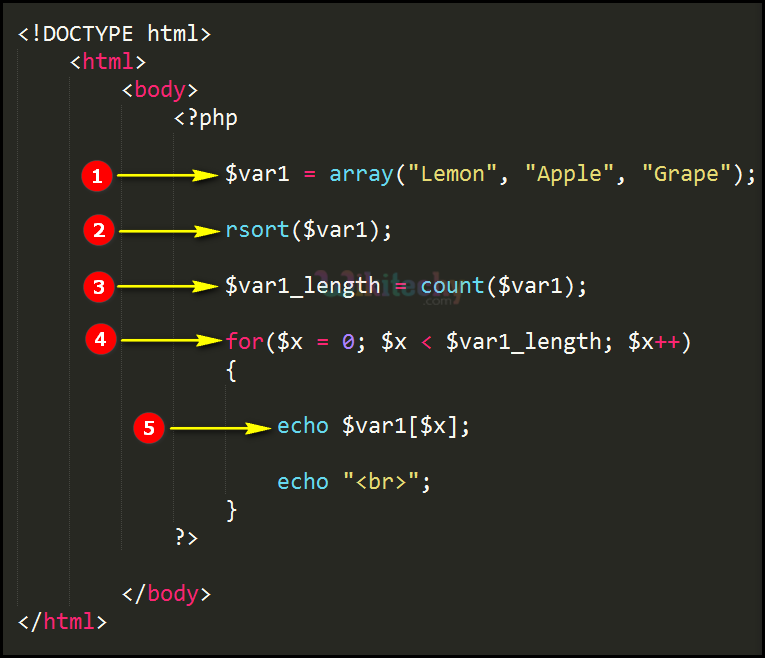# php tutorial - PHP rsort Characters - php programming - learn php - php code - php script

• In php the rsort function is used for sorting the array in terms of descending order.
• Basically the characters are reverse sorted using rsort function in terms of alphabets order from Z to A Format.

## php program Code Explanation :1. In this statement “\$var1” is an array variable having array values “Lemon, Apple and Grape”.
2. sort(\$var1); is the Sort function called over here which is sorting the array values (Lemon,Apple and Grape) in descending order.
3. In this statement the count function is used for counting the number of values in the array variable “var1” which is being stored in variable \$var1_length .
4. Statement defining the for loop statement for processing the array values.
5. echo \$var1[\$x]; This echo statement will print the sorted array values from the variable “\$var1”.

## php coding Sample Output :1. Output of the above php sort code shows the php array values (Lemon, Apple and Grape) sorted in descending order (Lemon, Grape and Apple) as shown here.